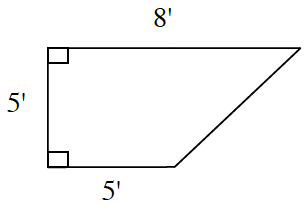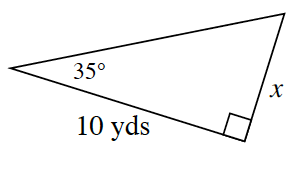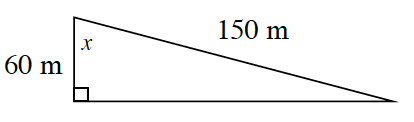### Home > CCG > Chapter 7 > Lesson 7.3.2 > Problem7-136

7-136.This problem is a checkpoint for solving with trigonometric ratios and the Pythagorean Theorem. It will be referred to as Checkpoint 7.

1. Compute the perimeter.1. Solve for $x$.1. Solve for $x$.2. Juanito is flying a kite at the park and realizes that all $500$ feet of string are out. Margie measures the angle of the string with the ground using her clinometer and finds it to be $42°$. How high is Juanito’s kite above the ground? Draw a diagram and use the appropriate trigonometric ratio.

Check your answers by referring to the Checkpoint 7 materials located at the back of your book.

Ideally, at this point you are comfortable working with these types of problems and can solve them correctly. If you feel that you need more confidence when solving these types of problems, then review the Checkpoint 7 materials and try the practice problems provided. From this point on, you will be expected to do problems like these correctly and with confidence.

Answers and extra practice are located in the back of your printed textbook or in the Reference Tab of your eBook.
If you have an eBook for CCG, login and then click the following link: Checkpoint 7: Solving with Trigonometric Ratios and the Pythagorean Theorem